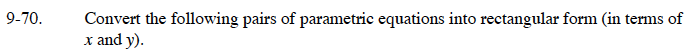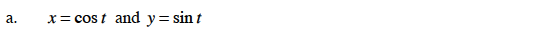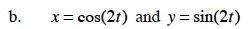### Home > CALC > Chapter 9 > Lesson 9.2.2 > Problem9-70

9-70.
1. Convert the following pairs of parametric equations into rectangular form (in terms of x and y). Homework Help ✎

1. x = cos t and y = sin t

2. x = cos(2t) and y = sin(2t)

3. x = t4 − 3t2 and y = t2sin2(x) + cos2(x) = 1